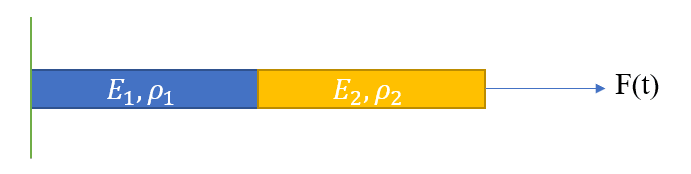×
INTELLIGENT WORK FORUMS
FOR ENGINEERING PROFESSIONALS

Are you an
Engineering professional?
Join Eng-Tips Forums!
• Talk With Other Members
• Be Notified Of Responses
• Keyword Search
Favorite Forums
• Automated Signatures
• Best Of All, It's Free!

*Eng-Tips's functionality depends on members receiving e-mail. By joining you are opting in to receive e-mail.

#### Posting Guidelines

Promoting, selling, recruiting, coursework and thesis posting is forbidden.

# How do I solve cantilever bar made up of two materials analytically (dynamic analysis)?

 Forum Search FAQs Links MVPs

## How do I solve cantilever bar made up of two materials analytically (dynamic analysis)?

(OP)
Hello Eng-Tips team,

I am trying to solve the below problem analytically∇∙σ + 𝑏_𝑥 = 𝜌 (𝜕^2 𝑢)/(𝜕𝑡^2 ) --> (1) is the governing equation
[Note: In the above problem, b_x = 0 (no body forces)]
IC: u(x,0) = v(x,0) = 0
BC: u(0,t) =0; u'(x=1,t) = F(t)/(A*E2)
[Note: Since force (F(t)) is applied on bar that has modulus E2, I'm assuming the BC is F(t)/(A*E2)]].

I'm having trouble understanding how to solve the above problem.

Q1). Does interface conditions come into play in this problem? The displacements, stresses are continuous at the interface (Assuming it is perfectly bonded).
Q2). If I consider each portion of the bar individually (material1 or material2), how do the boundary conditions behave?

Any help would be much appreciated. Thank you!

### RE: How do I solve cantilever bar made up of two materials analytically (dynamic analysis)?

Not sure what you're trying to solve for, but I can tell you this much - F(t) is constant through both sections, so the displacement of each part is (F(t)/ A*E)*L, where L is the length of the section under consideration. The total displacement is the sum of the displacements of the 2 parts. I hope that helps.

Rod Smith, P.E., The artist formerly known as HotRod10

### RE: How do I solve cantilever bar made up of two materials analytically (dynamic analysis)?

(OP)
Hello BridgeSmith,

Thank you for the response. In this problem I'm solving for displacement only.

1. The blue color section is fixed at the left end. So, the BC is u1(x=0,t) = 0.
2. Now, if I consider the yellow section the left boundary is the right boundary of the blue section.
So, can we say u2(x=0.5,t) = u1(x=0.5, t)?

Note: u1 is the displacement of section 1,u2 is the displacement of section 2.

When I consider each of the above sections separately, I have a differential equation that I need to solve. (Governing equation (1) mentioned in the problem).
This governing equation could be solved if I impose 2 Initial and 2 Boundary conditions.
i.e,

For blue section:
∇∙σ1 + 𝑏_𝑥 = 𝜌1 (𝜕^2 𝑢1)/(𝜕𝑡^2 ) --> (1) is the governing equation
[Note: In the above problem, b_x = 0 (no body forces)]
IC: u1(x,0) = v1(x,0) = 0
BC: u1(0,t) =0; u1'(x=1,t) = ?
Note: Based of your first answer, u1'(x=0.5,t) = F(t)/(A*E1)

For yellow section:
∇∙σ2 + 𝑏_𝑥 = 𝜌2 (𝜕^2 𝑢2)/(𝜕𝑡^2 ) --> (1) is the governing equation
[Note: In the above problem, b_x = 0 (no body forces)]
IC: u2(x,0) = v2(x,0) = 0
BC: u2(0.5,t) =?; u2'(x=1,t) = F(t)/(A*E2)

Is this u2(0.5,t) = u1(0.5,t)?

I was majorly confused about how to handle the boundary conditions.

#### Red Flag This Post

Please let us know here why this post is inappropriate. Reasons such as off-topic, duplicates, flames, illegal, vulgar, or students posting their homework.

#### Red Flag Submitted

Thank you for helping keep Eng-Tips Forums free from inappropriate posts.
The Eng-Tips staff will check this out and take appropriate action.

#### Posting in the Eng-Tips forums is a member-only feature.

Click Here to join Eng-Tips and talk with other members! Already a Member? Login

#### Resources

Low-Volume Rapid Injection Molding With 3D Printed Molds
Learn methods and guidelines for using stereolithography (SLA) 3D printed molds in the injection molding process to lower costs and lead time. Discover how this hybrid manufacturing process enables on-demand mold fabrication to quickly produce small batches of thermoplastic parts. Download Now
Design for Additive Manufacturing (DfAM)
Examine how the principles of DfAM upend many of the long-standing rules around manufacturability - allowing engineers and designers to place a partâ€™s function at the center of their design considerations. Download Now
Industry Perspective: Education and Metal 3D Printing
Metal 3D printing has rapidly emerged as a key technology in modern design and manufacturing, so itâ€™s critical educational institutions include it in their curricula to avoid leaving students at a disadvantage as they enter the workforce. Download Now

Close Box

# Join Eng-Tips® Today!

Join your peers on the Internet's largest technical engineering professional community.
It's easy to join and it's free.

Here's Why Members Love Eng-Tips Forums:

•Talk To Other Members
• Notification Of Responses To Questions
• Favorite Forums One Click Access
• Keyword Search Of All Posts, And More...

Register now while it's still free!